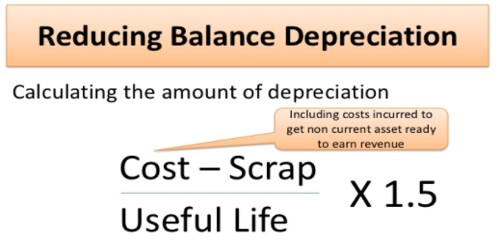Accounting

# Reducing Balance Method for Calculating DepreciationDiminishing Balance Method or Reducing Balance Method for Calculating Depreciation

Depreciation is a decrease in the price of an asset with the course of time, due in particular to wear and tear. All assets whose advantage is derived for a long period of time, generally more than one year period are called as Fixed Assets.

Under Reducing Balance Method, depreciation is charged at a fixed percentage each year on the reducing balance (i.e., cost less depreciation) of asset. The amount of depreciation goes on decreasing every year. For example, if the asset is purchased for Rs.1,00,000 and depreciation is to be charged at 10% p.a. on reducing balance method, then

Depreciation for the 1st year = 10% on Rs.1,00,000, ie., Rs.10,000

Depreciation for the 2nd year = 10% on Rs.90,000 (Rs.1,00,000 – Rs.10,000)

= Rs. 9,000

Depreciation for the 3rd year = 10% on Rs.81,000 (Rs.90,000 – Rs.9,000)

= Rs.8,100 and so on.

Merits:

• Uniform effect on the Profit and Loss account of different years: The total charge (i.e., depreciation plus repairs and renewals) remains almost uniform year after year, since in earlier years the amount of depreciation is more and the amount of repairs and renewals is less, whereas in later years the amount of depreciation is less and the amount of repairs and renewals is more.
• Recognized by the Income Tax authorities: This method is recognised by the Income Tax authorities
• Logical Method: It is a logical method as the depreciation is calculated on the diminished balance every year.

Demerits:

It is very difficult to determine the rate by which the value of asset could be written down to zero.